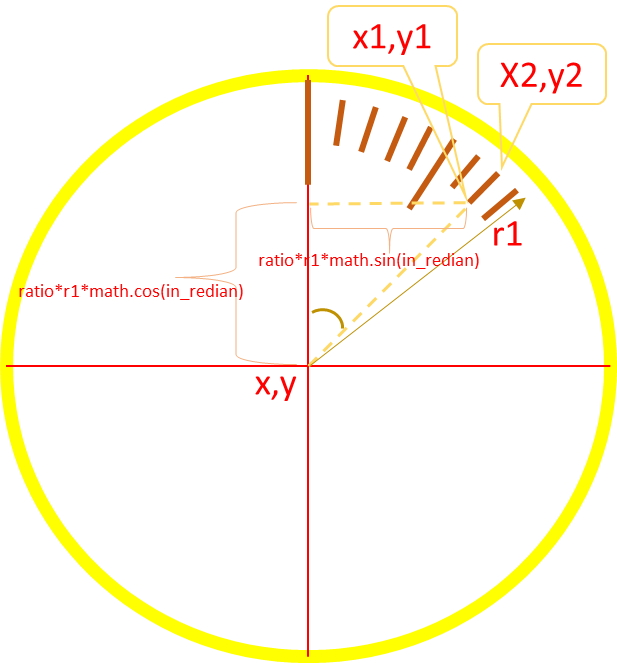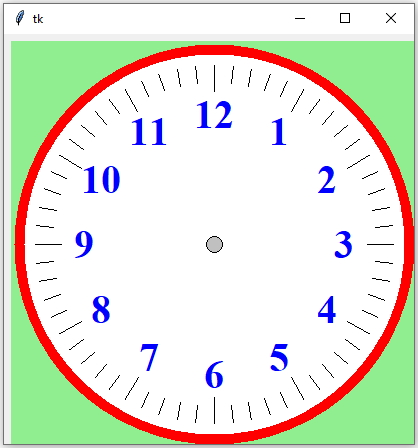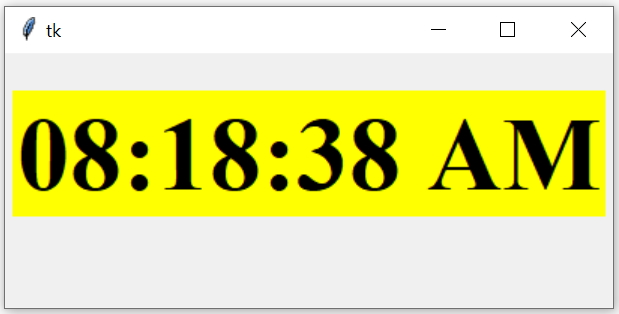# Analog Clock Dial``````    x1=x+ratio*r1*math.sin(in_radian)
Drawing Analog clock on Tkinter Canvas by showing dial and hour numbers and minute segment markings
variablesDetails
r1:distance from center ( x, y) to outer edge of the lines ( each line shows one second or one minute )
ratiofraction of full length ( of r1 ) to get x2 and y2. Higer ratio mean small length of lines. This length is more ( less value of ratio ) for all lines after 5 intervals.
x,yCenter coordinates. Here x = fraction of full length ( of r1 ) to get x2 and y2. Higer ratio mean small length of lines. This length is more ( less value of ratio ) for all lines after 5 intervals.
x1,y1Inner edge coordinates of segment lines.
x2,y2Outer edge coordinates of segment lines.
in_degreeAngle in degree. Each segment is 6 degree so to draw the next line the value of in_degree has to be incremented by 6
in_radianInputs to sin() and cos() functions value in radian. Value in degree is converted to radian before using with sin() or cos() functions.

## Segments in circle

We have to draw 60 segments within 360 degrees or full circle. Each segment will be drawn after 6 degree of previous segment. Our for loop will start from 0 and will continue till 59. In each step there will be an increment of 6 degree.
``````for i in range(0,60):
.....
.....
in_degree=in_degree+6 # increment for next segment
``````

Before using the angle value as input to sin() or cos() functions we have to convert them to radian.
``in_radian = math.radians(in_degree) # converting to radian``
Inside our clock we can see that each 5th segment is longer than the other segments. For this we will change the ratio value for each fifth segment.
``````if(i%5==0):
ratio=0.85 # Long marks ( lines )
c1.create_text(t1,t2,fill='blue',font="Times 30  bold",text=next(h)) # number added
else:
ratio=0.9 # small marks ( lines )``````

## Adding text for each hours

One iterater object h is created with all numbers and each iteration one number is collected and displayed. As we are staring from 0 angle so the first element is 12.
``h=iter(['12','1','2','3','4','5','6','7','8','9','10','11'])``
We will be adding hour numbers to each 5th segment. This number is displayed at a distance r2 from the center of the clock. Here the style of the text can be changed by using font options.
``````    if(i%5==0):
ratio=0.85 # Long marks ( lines )
c1.create_text(t1,t2,fill='blue',font="Times 30  bold",text=next(h)) # number added
else:
ratio=0.9 # small marks ( lines )
``````Full code is here
``````import math
import tkinter as tk
import time

my_w = tk.Tk()
#my_w.tk.call('tk', 'scaling',200)
width,height=410,410 # set the variables
c_width,c_height=width-5,height-5 # canvas width height
d=str(width)+"x"+str(height)
my_w.geometry(d)
c1 = tk.Canvas(my_w, width=c_width, height=c_height,bg='lightgreen')
dial=c1.create_oval(10, 10, 400, 400,width=10,outline='#FF0000',fill='#FFFFFF')
x,y=205,205 # center
x1,y1,x2,y2=x,y,x,10 # second needle
center=c1.create_oval(x-8,y-8,x+8,y+8,fill='#c0c0c0')
r1=180 # dial lines for one minute
r2=130 # for hour numbers  before the lines
in_degree = 0
h=iter(['12','1','2','3','4','5','6','7','8','9','10','11'])
for i in range(0,60):
if(i%5==0):
ratio=0.85 # Long marks ( lines )
c1.create_text(t1,t2,fill='blue',font="Times 30  bold",text=next(h)) # number added
else:
ratio=0.9 # small marks ( lines )

c1.create_line(x1,y1,x2,y2,width=1) # draw the line for segment
in_degree=in_degree+6 # increment for next segment

my_w.mainloop()``````Subscribe to our YouTube Channel here

## Subscribe

* indicates required
Subscribe to plus2netplus2net.com